• # 量子物理

## 第一章 光的特性

### 1.2 几何光学与费马原理

#### 1.2.1. 几何光学的基本定律

##### 3. 光的反射和折射定律
###### (1) 反射定律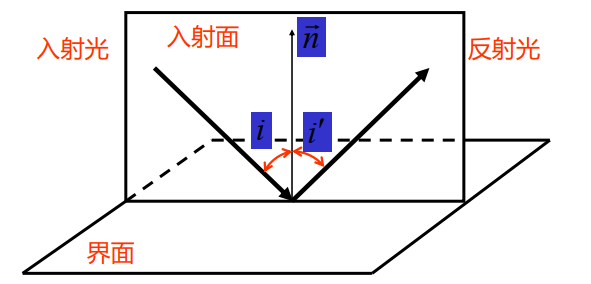• 反射光在入射面内；

• 反射角等于入射角，

${i}^{\prime }=i.$
###### (2) 折射定律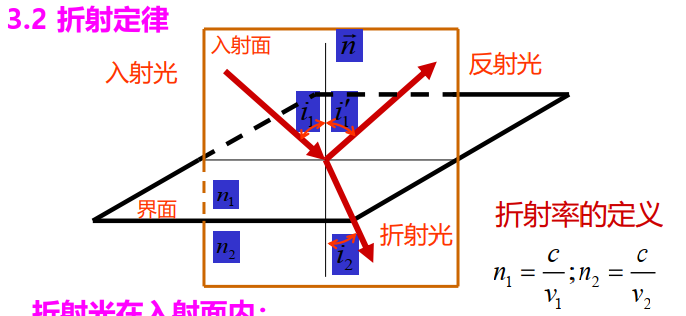折射率的定义：

${n}_{1}=\frac{c}{{v}_{1}},{n}_{2}=\frac{c}{{v}_{2}}.$

折射率 $n$$n$ 大的介质称为光密介质，小的称为光疏介质.

• 折射光在入射面内；

• 入射角的正弦与折射角的正弦之比，对于给定介质及光波长，是一个常数，

有斯涅尔（Snell）定律

${n}_{1}\mathrm{sin}{i}_{1}={n}_{2}\mathrm{sin}{n}_{2}.$

重要例子：全反射原理，当光从光密介质射到光疏介质表面时，可能会出现全反射现象.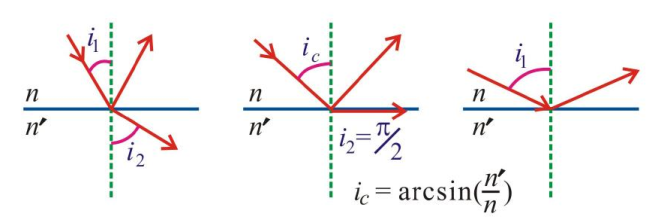#### 1.2.2 费马原理

光程：光在均匀介质中通过的几何路径 $s$$s$ 与所经过的介质折射率 $n$$n$ 的乘积，

$l=ns.$

光在介质中走过的光程等于以相同的时间在真空中走过的距离，即

$t=\frac{s}{v}=\frac{s}{c/n}=\frac{l}{c}.$

费马原理的表述：

• 空间两点间的实际光线路径是光程为平稳值的路径. 这里，”平稳“指的是当光线以任何方式对该路径有无限小的偏离时，相应光程的一阶改变量为零，即

• 空间中两点的实际光线路径，与其他相邻的可能路径相比较，其光程（或传播时间）取极值.

因此，费马原理也称为光程极值原理或时间极值原理. 多数情况下，指最小值，故该原理又曾称为最小时间（或光程）原理.

意义：描述光线传播行为的普遍规律.

例子：直线传播定律，

• 反射定律

• 折射定律

#### 1.2.3 光学成像

##### 1. 薄透镜近似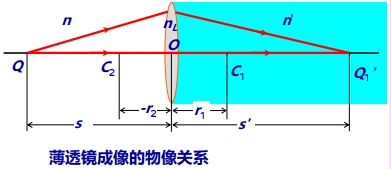薄透镜成像的物像关系：

$\frac{n}{s}+\frac{{n}^{\prime }}{{s}^{\prime }}=\frac{{n}_{L}-n}{{r}_{1}}+\frac{{n}^{\prime }-{n}_{L}}{{r}_{2}},$

其中 ${r}_{1},{r}_{2}$$r_1,r_2$ 分别为两个球面的半径.

##### 2. 薄透镜的焦点与焦平面

${s}^{\prime }=\mathrm{\infty }$$s'=\infty$，得物方焦距

$f=\frac{n}{\frac{{n}_{L}-n}{{r}_{1}}+\frac{{n}^{\prime }-{n}_{L}}{{r}_{2}}}=\frac{n}{\mathrm{\Phi }}.$

$s=\mathrm{\infty }$$s=\infty$，得像方焦距

${f}^{\prime }=\frac{{n}^{\prime }}{\frac{{n}_{L}-n}{{r}_{1}}+\frac{{n}^{\prime }-{n}_{L}}{{r}_{2}}}=\frac{{n}^{\prime }}{\mathrm{\Phi }}.$

空气中的薄透镜：$n={n}^{\prime }=1$$n=n'=1$，有磨镜者公式

$f={f}^{\prime }=\frac{1}{\left({n}_{L}-1\right)\left(\frac{1}{{r}_{1}}-\frac{1}{{r}_{2}}\right)}.$
##### 3. 高斯物像公式
$\begin{array}{c}\frac{{f}^{\prime }}{{s}^{\prime }}+\frac{f}{s}=1,\\ \frac{f}{{f}^{\prime }}=\frac{n}{{n}^{\prime }}.\end{array}$

### 1.3 光是电磁波

#### 单色光波及其描述

单色光波的四个条件：

1. 空间格点的电磁场以同一频率做简谐振荡；

2. 各点的振幅不随着时间变化；

3. 初相位是空间分布，与时间无关；

4. 波列在空间上无限延伸，在时间上无限长.

在均匀介质中，沿 $z$$z$ 轴正向传播，以速度 $v$$v$ 传播的平面简谐电磁波为

$\begin{array}{c}\stackrel{\to }{E}={\stackrel{\to }{E}}_{0}\mathrm{cos}\left[\omega \left(t-\frac{z}{v}\right)+{\phi }_{0}\right],\\ \stackrel{\to }{B}={\stackrel{\to }{B}}_{0}\mathrm{cos}\left[\omega \left(t-\frac{z}{v}\right)+{\phi }_{0}\right].\end{array}$

波面（等相面）：波场中相位相同的点的集合.

平面单色波：波面是平面的单色波.

沿着任意方向传播：

$E={E}_{0}\mathrm{cos}\left(\omega t-\stackrel{\to }{k}\cdot \stackrel{\to }{r}\right),$

特别，若沿着 $+z$$+z$ 方向传播，则

$E={E}_{0}\mathrm{cos}\left(\omega t-kz\right).$

其中，

$\stackrel{\to }{k}=\frac{2\pi }{\lambda }\stackrel{\to }{\kappa }$

称为波矢，其方向指向波的传播方向.

#### 简谐波的复数表示式

$E\left(z,t\right)={E}_{0}{\mathrm{e}}^{-\mathrm{i}\left(\omega t-kz\right)}={E}_{0}{\mathrm{e}}^{\mathrm{i}kz}{\mathrm{e}}^{-i\omega t},$

包含时间变量和空间变量的两部分完全分离开.

$\stackrel{~}{E}={E}_{0}{\mathrm{e}}^{\mathrm{i}kz},\phantom{\rule{1em}{0ex}}\stackrel{~}{E}={E}_{0}\left(p\right){\mathrm{e}}^{\mathrm{i}\varphi \left(p\right)}$

称为复振幅. 其模量 $A$$A$ 代表振幅在空间的分布，其辐角代表相位在空间的分布.

• 平面波的复振幅

$\stackrel{~}{E}={E}_{0}{\mathrm{e}}^{\mathrm{i}\stackrel{\to }{k}\cdot \stackrel{\to }{r}}.$
• 球面波的复振幅

$\stackrel{~}{E}=\frac{A}{r}{\mathrm{e}}^{\mathrm{i}\stackrel{\to }{k}\cdot \stackrel{\to }{r}}.$

### 1.4 光的干涉

#### 发生条件

• 必要条件：

• 频率相同（适用于所有波动）；

• 振动方向一致或有平行的振动分量（仅适用于矢量波）；

• 相位差在观察时间内稳定分布（尤为重要）；

• 充分条件：参与相干的光振幅大致差不多.

#### 杨氏双缝干涉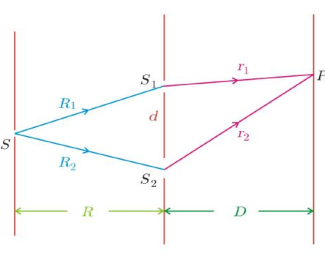相邻两条明（暗）条纹的间距

$\mathrm{\Delta }x=\frac{D}{d}\lambda ,$

其中 $D$$D$ 为双缝到屏间距，$d$$d$ 为双缝间距. 条纹间距相等，与级数无关.

#### 薄膜干涉

##### 等厚干涉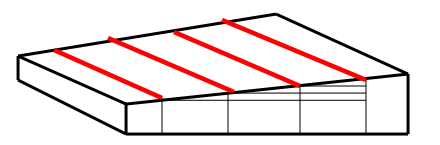垂直入射，厚度相等的地方，是同一级亮条纹，故称等厚干涉.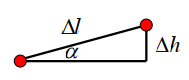相邻条纹的厚度差

$2{n}_{2}\mathrm{\Delta }h=\lambda ,$

条纹间距

$\mathrm{\Delta }l=\frac{\mathrm{\Delta }h}{\mathrm{sin}\alpha }=\frac{\lambda }{2{n}_{2}\mathrm{sin}\alpha },$

即干涉条纹是一系列等间距的平行直条纹.

##### 牛顿环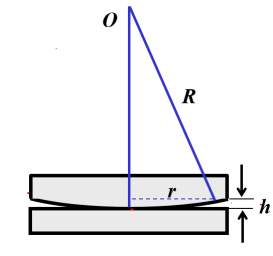高度

${h}_{m}=R-\sqrt{{R}^{2}-{r}_{m}^{2}}=\frac{{r}_{m}^{2}}{2R}.$

$R\gg {r}_{m}$$R\gg r_m$，即 $\frac{{r}_{m}}{R}\to 0$$\dfrac{r_m}{R}\to0$. 借助 $\left(1+x{\right)}^{\alpha }-1\sim \alpha x\phantom{\rule{1em}{0ex}}\left(x\to 0\right)$$(1+x)^\alpha-1\sim\alpha x\quad(x\to 0)$ 即得.

• 亮条纹的位置：

${h}_{m}=\left(m+\frac{1}{2}\right)\frac{\lambda }{2};$
• 暗条纹的位置：

${h}_{m}^{\prime }=\frac{m\lambda }{2};$
• 明环半径：

${r}_{m}=\sqrt{\left(m+\frac{1}{2}\right)R\lambda };$
• 暗环半径：

${r}_{m}^{\prime }=\sqrt{mR\lambda }.$

图样特点：

• 干涉图样圆环半径越大，相应的干涉级越高；

• 间距不等，随着圆环半径增大，条纹变密；

• 从反射光中观测，牛顿环的中心点是暗点；从透射光中观测，牛顿环的中心点是亮点.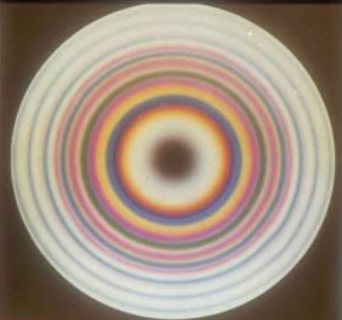应用：测量透镜的曲率半径.

测出任意两级暗环的半径（或直径），数出它们的级数差 $N$$N$，则透镜的曲率半径

$R=\frac{{r}_{m+N}^{2}-{r}_{m}^{2}}{N\lambda }=\frac{{D}_{m+N}^{2}-{D}_{m}^{2}}{4N\lambda }.$

$R$$R$ 已知，可以测量照射光的波长

$\lambda =\frac{{D}_{m+N}^{2}-{D}_{m}^{2}}{4NR}.$
##### 增透膜和增反膜

利用薄膜干涉提高光学器件的透光率.

### 1.5 光的衍射

#### 特点

• 衍射效应与某种复杂的干涉效应有关系；

• 限制与扩展；

• 衍射效应的强弱与障碍物线度 $\rho$$\rho$ 与光波长 $\lambda$$\lambda$ 之比关系十分密切：

• $\rho \sim {10}^{3}\lambda$$\rho\sim10^3\lambda$ 以上，衍射效应不明显；

• $\rho \sim {10}^{3}\lambda -10\lambda$$\rho\sim 10^3\lambda-10\lambda$，衍射效应明显；

• $\rho ⩽\lambda$$\rho\leqslant\lambda$，向散射过渡.

#### 巴比涅原理

互补屏产生的衍射场复振幅之和等于自由传播时场的复振幅，即

${\stackrel{~}{E}}_{a}\left(P\right)+{\stackrel{~}{E}}_{b}\left(P\right)={\stackrel{~}{E}}_{0}\left(P\right).$

两互补屏在后焦面上产生的夫琅禾费衍射强度完全相同（像点除外）.

#### 夫琅禾费圆孔衍射

艾里斑

• 半角宽度（角分辨极限）

$\mathrm{\Delta }\theta =1.22\frac{\lambda }{D},$

其中 $D=2a$$D=2a$ 是圆孔直径.

• 半径（线分辨极限）

$\mathrm{\Delta }l=f\mathrm{\Delta }\theta =1.22\frac{\lambda f}{D}.$

### 1.6 光的偏振

#### 分类

• 线偏振光

• 圆偏振光

• 椭圆偏振光

• 自然光

• 部分偏振光

偏振度

$P=\frac{{I}_{\mathrm{max}}-{I}_{\mathrm{min}}}{{I}_{\mathrm{max}}+{I}_{\mathrm{min}}}.$
• $P=1$$P=1$：线偏振光；

• $0$0：部分偏振光或椭圆偏振光；

• $P=0$$P=0$：自然光或圆偏振光

#### 偏振光的合成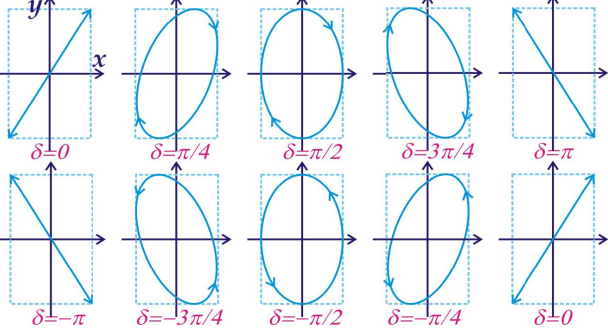#### 偏振片对不同偏振态的光强响应

线偏振光：马吕斯定律，

$I={I}_{0}{\mathrm{cos}}^{2}\alpha .$

#### 光的散射

应用：

• 自然光经散射，一般将变为部分偏振光，雨后初晴出现的虹和霓也是部分偏振光；

• 直视太阳，进入眼睛的主要是被大气散射之后透射过来的光；

• 巡视天空，进入眼睛的是阳光经大气散射之后的光；

• 蓝天，瑞利散射；

• 白云，米·德拜散射.

### 1.7 黑体辐射

#### Stefan-Boltzmann 定律

辐射的总能量（曲线下的面积）与 ${T}^{4}$$T^4$ 成正比，

其中 $\sigma$$\sigma$ 是 Stefan-Boltzmann 常数.

#### Wien 位移定律

曲线的极大值满足

$T{\lambda }_{m}=b,$

其中 $b=2.8978\times10^{-3}\ \mathrm{mK}$.

用于测量温度，

$T=\frac{b}{{\lambda }_{m}}.$

#### Rayleigh-Jeans 定律

电磁学和统计物理严格求解得

$E\left(\lambda ,T\right)=\frac{2\pi c}{{\lambda }^{4}}kT.$

#### 普朗克能量子假说

空腔中的驻波是一系列的谐振子，只能取一些分立的能量，

$\epsilon =0,{\epsilon }_{0},2{\epsilon }_{0},\cdots ,$

其中 ${\epsilon }_{0}=h\nu$$\varepsilon_0=h\nu$$h=6.63\times10^{-34}\ \mathrm{J\cdot s}$.

黑体的辐射本领为

$E\left(\nu ,T\right)=\frac{2\pi h}{{c}^{2}}\frac{{\nu }^{3}}{{\mathrm{e}}^{\frac{h\nu }{kT}}-1}.$

### 1.8 光电效应

#### 光电效应的解释

光子的能量

$\epsilon =h\nu ;$

光电效应方程

$h\nu =\frac{1}{2}m{v}^{2}+A,$

$A$$A$ 为电子从金属表面逸出所需的逸出功.

#### 光的波粒二象性

• 光子能量：

$\epsilon =h\nu ;$
• 光子质量

$m=\frac{h\nu }{{c}^{2}};$
• 光子动量

$p=\frac{E}{c}=\frac{h\nu }{c}=\frac{h}{\lambda };$
• 粒子性：一个光子是一个不可分割的主体；

• 波动性：具有波的特征，

$\lambda =\frac{h}{p}.$

光同时具有粒子性和波动性.

#### de Broglie 的物质波

• 所有的波都具有粒子性；

• 所有的粒子都具有波动性；

• $\lambda =h/p$$\lambda=h/p$

• $p=mv/\sqrt{1-{v}^{2}/{c}^{2}}$$p=mv/\sqrt{1-v^2/c^2}$

• 不能将物质的运动和波的传播分开.

## 第二章 波尔原子模型

### 2.3 波尔氢原子理论

#### 2.3.2 波尔氢原子模型

##### 1. 波尔假设
• 定态假设：原子的能量状态是分立的，电子只在某些特定的轨道上运动，每个轨道对应一个定态 ${E}_{n}$$E_n$，即使电子在这些轨道上做加速运动，也不向外辐射能量. 其中，

${E}_{n}=-\frac{1}{2}\frac{Z{e}^{2}}{4\pi {\epsilon }_{0}{r}_{n}};$
• 角动量量子化假设：电子处于上述 定态时，角动量是量子化的，

$L={m}_{e}rv=n\hslash ,\phantom{\rule{1em}{0ex}}\hslash =h/2\pi ;$
• 频率条件：当电子吸收或放出能量时，它会在不同轨道间发生跃迁，跃迁前后的能量差满足

$\nu =|{E}_{n}-{E}_{m}|/h.$
##### 2. 波尔模型
###### (1) （类）氢原子的大小

则量子化的轨道半径为

${r}_{n}={a}_{0}\cdot \frac{{n}^{2}}{Z},$

相应的轨道速率为

${v}_{n}=\frac{Z\alpha c}{n},$

其中精细结构常数

$\alpha =\frac{{e}^{2}}{4\pi {\epsilon }_{0}\hslash c}\approx \frac{1}{137}.$

$Z=1$$Z=1$ 时，氢原子的

• 轨道半径 ${r}_{n}={n}^{2}{a}_{0}$$r_n=n^2a_0$. 特别，第一轨道半径 ${r}_{1}={a}_{0}$$r_1=a_0$

• 波尔速度 ${v}_{n}=\alpha c/n$$v_n=\alpha c/n$. 特别，第一波尔速度 ${v}_{1}={e}^{2}/\left(4\pi {\epsilon }_{0}\hslash \right)=\alpha c$$v_1=e^2/(4\pi\varepsilon_0\hbar)=\alpha c$.

###### (2) （类）氢原子的定态能量——量子化的波尔能级
${E}_{n}=-hcR\frac{{Z}^{2}}{{n}^{2}},$

其中 $R$$R$ 为里德伯常量. 当 $Z=1,n=1$$Z=1,n=1$ 时就是氢原子的基态

$n⩾2$$n\geqslant2$ 的状态称为原子的激发态，

###### (3) （类）氢原子的光谱

光谱公式

$\stackrel{~}{\nu }=R{Z}^{2}\left(\frac{1}{{m}^{2}}-\frac{1}{{n}^{2}}\right),\phantom{\rule{1em}{0ex}}\stackrel{~}{\nu }=\frac{\nu }{c}=\frac{1}{\lambda }.$

$Z=1$$Z=1$ 时即为里德伯方程

$\stackrel{~}{\nu }=R\left(\frac{1}{{m}^{2}}-\frac{1}{{n}^{2}}\right),$

其中里德伯常量

#### 2.3.3 波尔原子模型的问题

• 经典和量子并存；

• 预言了发射和吸收光子的频率，但是光谱线的强度、极化、选择定则不能解释；

• 无法解释更复杂的光谱现象.

## 第三章 波函数与测量

### 3.2 波函数的物理意义

#### 3.2.1 定义

$\psi \left(\mathbit{x},t\right)={\psi }_{0}{\mathrm{e}}^{\mathrm{i}\left(\mathbit{k}\cdot \mathbit{x}-\omega t\right)}={\psi }_{0}{\mathrm{e}}^{\mathrm{i}\left(\mathbit{p}\cdot \mathbit{x}-Et\right)/\hslash },$

其中 $\mathbit{x}=x{\mathbit{e}}_{x}+y{\mathbit{e}}_{y}+z{\mathbit{e}}_{z}$$\boldsymbol x=x\boldsymbol e_x+y\boldsymbol e_y+z\boldsymbol e_z$ 为位矢，$\mathbit{k}={k}_{x}{\mathbit{e}}_{x}+{k}_{y}{\mathbit{e}}_{y}+{k}_{z}{\mathbit{e}}_{z}$$\boldsymbol k=k_x\boldsymbol e_x+k_y\boldsymbol e_y+k_z\boldsymbol e_z$ 为波矢，$\mathbit{p}={p}_{x}{\mathbit{e}}_{x}+{p}_{y}{\mathbit{e}}_{y}+{p}_{z}{\mathbit{e}}_{z}$$\boldsymbol p=p_x\boldsymbol e_x+p_y\boldsymbol e_y+p_z\boldsymbol e_z$ 为动量.

波的强度或振幅反映的是粒子在时刻 $t$$t$、空间点 $P$$P$ 处出现或被发现的几率或几率幅.

#### 3.2.2 对波函数的要求

• 单值

• 有限

• 连续

• 粒子不能湮灭，即总能在空间某处发现该粒子，

#### 3.2.3 波函数的归一化条件

其中 $1/\sqrt{A}$$1/\sqrt A$ 称为归一化因子.

#### 3.2.4 态与态叠加原理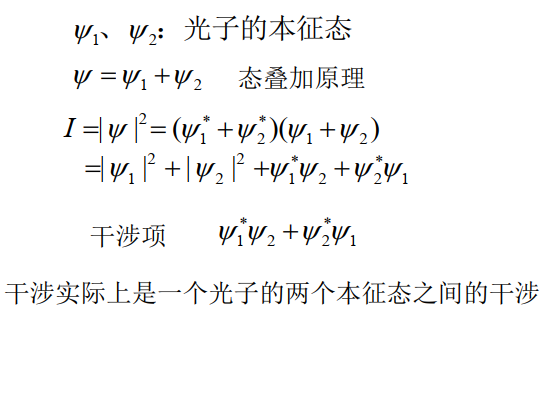### 3.3 不确定关系

#### 3.3.1 海森堡不确定性原理（测不准原理）

若两个力学量 $p$$p$$q$$q$ 不对易，即 $pq-qp\ne 0$$pq-qp\neq0$，则 $p$$p$$q$$q$ 不能同时具有可确定的值，不确定程度

$\mathrm{\Delta }p\mathrm{\Delta }q⩾\frac{\hslash }{2}.$

#### 3.3.2 物理含义

• 物质不可能同时具有确定的空间位置和动量；

• 能级的自然宽度，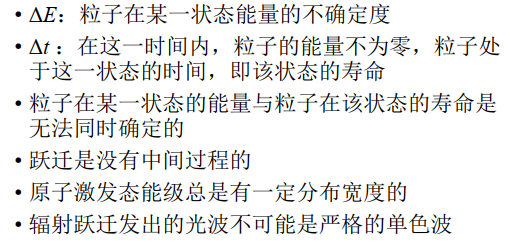• 束缚粒子的平均最小动能，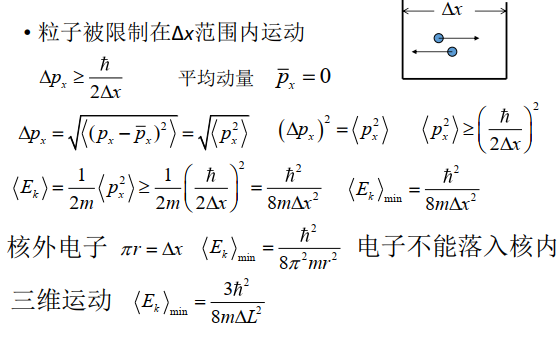### 3.4 薛定谔方程

• 必须满足德布罗意关系式

$E=h\nu =\hslash \omega ,\phantom{\rule{1em}{0ex}}p=\frac{h}{\lambda }=\hslash k;$
• 条件：没有粒子的产生和湮灭现象，速度足够小；

• 薛定谔波动方程描述了一个质量为 $m$$m$ 的粒子在势场 $V\left(\mathbit{r},t\right)$$V(\boldsymbol r,t)$ 中运动状态随时间的变化，知道 $\psi \left(\mathbit{r},0\right)$$\psi(\boldsymbol r,0)$ 可求出 $\psi \left(\mathbit{r},t\right)$$\psi(\boldsymbol r,t)$

• $\psi$$\psi$ 是复数，本身不代表什么物理含义，$|\psi {|}^{2}$$|\psi|^2$ 是实数，代表概率密度.

自由粒子的薛定谔方程

$\mathrm{i}\hslash \frac{\partial \psi \left(\mathbit{x},t\right)}{\partial t}=-\frac{{\hslash }^{2}}{2m}{\mathrm{\nabla }}^{2}\psi \left(\mathbit{x},t\right);$

对于处在势场 $V\left(\mathbit{x},t\right)$$V(\boldsymbol x,t)$ 中的粒子，

$\mathrm{i}\hslash \frac{\partial \psi \left(\mathbit{x},t\right)}{\partial t}=\left[-\frac{{\hslash }^{2}}{2m}{\mathrm{\nabla }}^{2}+V\left(\mathbit{x},t\right)\right]\psi \left(\mathbit{x},t\right);$

对于定态势能场 $V\left(\mathbit{x},t\right)=V\left(\mathbit{x}\right)$$V(\boldsymbol x,t)=V(\boldsymbol x)$，有定态薛定谔方程（Hamilton 方程），

$\left[-\frac{{\hslash }^{2}}{2m}{\mathrm{\nabla }}^{2}+V\left(\mathbit{x}\right)\right]\psi \left(\mathbit{x}\right)=E\psi \left(\mathbit{x}\right),$

其解为

$\psi \left(\mathbit{x},t\right)=\psi \left(\mathbit{x}\right){\mathrm{e}}^{-\mathrm{i}Et/\hslash }.$

### 3.5 力学量的算符表示

#### 平均值

此外，涨落

$\mathrm{\Delta }x=\sqrt{⟨{x}^{2}⟩-{⟨x⟩}^{2}}.$

#### 算符

$\begin{array}{c}\stackrel{^}{p}=-\mathrm{i}\hslash \mathrm{\nabla },\\ \stackrel{^}{H}=-\frac{{\hslash }^{2}}{2m}{\mathrm{\nabla }}^{2}+V\left(\mathbit{x},t\right),\\ \stackrel{^}{L}=\stackrel{^}{r}×\left(-\mathrm{i}\hslash \mathrm{\nabla }\right).\end{array}$

#### 本征函数与本征值

• Hamilton 量

$E=\frac{{p}^{2}}{2m}+V\left(\mathbit{x},t\right);$
• Hamilton 算符

$\stackrel{^}{H}=-\frac{{\hslash }^{2}}{2m}{\mathrm{\nabla }}^{2}+V\left(\mathbit{x},t\right);$
• Hamilton 方程

$\left[-\frac{{\hslash }^{2}}{2m}{\mathrm{\nabla }}^{2}+V\left(\mathbit{x}\right)\right]\psi \left(\mathbit{x}\right)=E\psi \left(\mathbit{x}\right),$

亦即

$\stackrel{^}{H}\psi \left(\mathbit{x}\right)=E\psi \left(\mathbit{x}\right).$

这里，$E$$E$$\stackrel{^}{H}$$\hat H$ 的本征值，$\psi \left(\mathbit{x}\right)$$\psi(\boldsymbol x)$$\stackrel{^}{H}$$\hat H$ 的本征函数，$\stackrel{^}{H}\psi \left(\mathbit{x}\right)=E\psi \left(\mathbit{x}\right)$$\hat H\psi(\boldsymbol x)=E\psi(\boldsymbol x)$ 是能量 $E$$E$ 的本征方程.

### 3.6 一维定态问题

1. 列出一维定态薛定谔方程

$\left[-\frac{{\hslash }^{2}}{2m}\frac{{\mathrm{d}}^{2}}{\mathrm{d}{x}^{2}}+V\left(x\right)\right]\psi \left(x\right)=E\psi \left(x\right);$
2. 根据波函数标准条件求出本征值 ${E}_{i}$$E_i$ 和本征函数 ${\psi }_{i}$$\psi_i$

• 边界条件

• 波函数连续

• 波函数一阶微商连续

• （非必须）归一化条件

3. 写出定态波函数即得到本征值 ${E}_{n}$$E_n$ 的定态波函数

${\psi }_{n}\left(x,t\right)={\psi }_{n}\left(x\right)\mathrm{exp}\left\{-\mathrm{i}{E}_{n}t/\hslash \right\};$
4. 通过归一化确定归一化系数 ${C}_{n}$$C_n$

## 第四章 氢原子

### 4.1 氢原子定态函数

#### 单原子电子的波函数

由库仑势

$V\left(r\right)=-\frac{Z{e}^{2}}{4\pi {\epsilon }_{0}r}$

得 Hamilton 算符

$\stackrel{^}{H}=-\frac{{\hslash }^{2}}{2m}{\mathrm{\nabla }}^{2}+V\left(r\right)=-\frac{{\hslash }^{2}}{2m}{\mathrm{\nabla }}^{2}-\frac{Z{e}^{2}}{4\pi {\epsilon }_{0}r},$

从而得到定态薛定谔方程